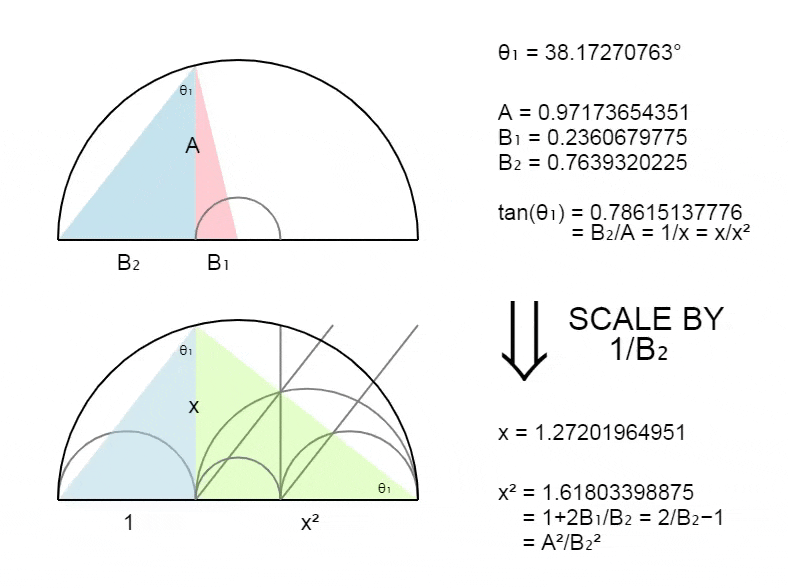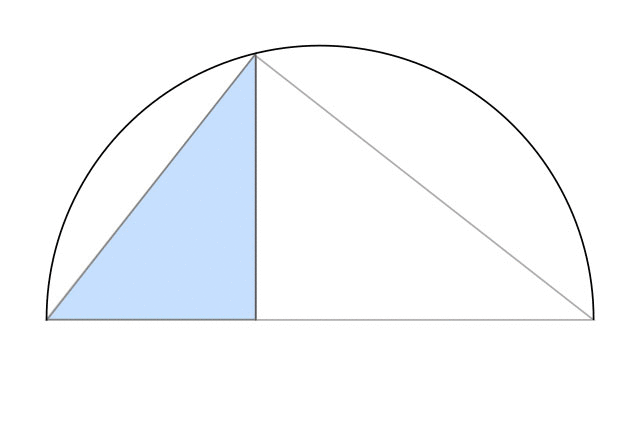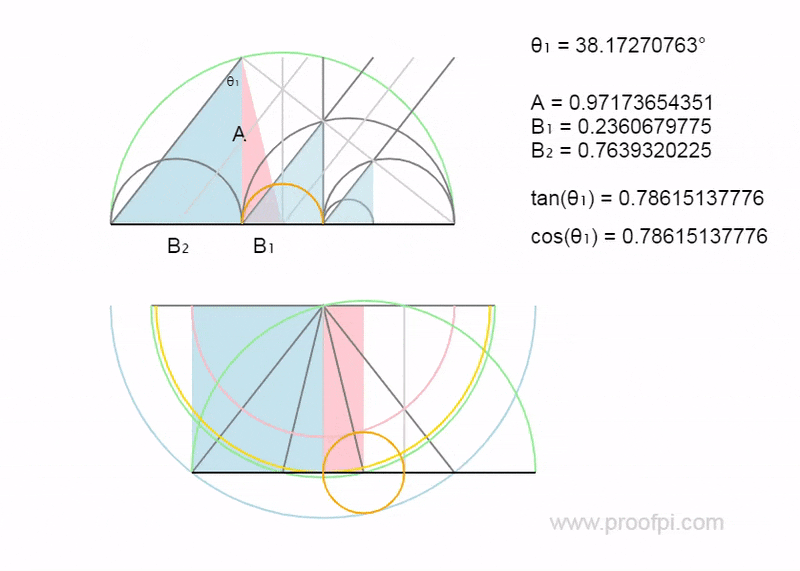## The Squared Circle

IF the circumference of a circle is equal to the perimeter of a square.ALL 3 points of the triangle are on the circle. cos(θ) = π/4.Animated example.The following is true.Substituting x for 4/π, as seen below.Substituting cos(θ₁) for π/4, as seen below.Simplifying.## The Problem

Lets explore.As we can see above, it seems the following must be true.Otherwise it will not have the following symmetry.These is only one angle with this symmetry, as seen in the animation below.To be continued..(last updated : 31/01/20)